# Introduction

This vignette demonstrates use of the basic functions of the Syuzhet package. The package comes with four sentiment dictionaries and provides a method for accessing the robust, but computationally expensive, sentiment extraction tool developed in the NLP group at Stanford. Use of this later method requires that you have already installed the coreNLP package (see http://nlp.stanford.edu/software/corenlp.shtml).

The goal of this vignette is to introduce the main functions in the package so that you can quickly extract plot and sentiment data from your own text files. This document will use a short example passage to demonstrate the functions and the various ways that the extracted data can be returned and or visualized.

## get_sentences

After loading the package (library(syuzhet)), you begin by parsing a text into a vector of sentences. For this you will utilize the get_sentences() function which implements the openNLP sentence tokenizer. The get_sentences() function includes an argument that determines how to handle quoted text. By default, quotes are stripped out before sentence parsing. (Thanks to Annie Swafford who observed that sentence parsing with openNLP improves when quotations are removed.) In the example that follows, a very simple text passage containing twelve sentences is loaded directly. (You could just as easily load a text file from your local hard drive or from a URL using the get_text_as_string() function. get_text_as_string() is described below.)

library(syuzhet)
my_example_text <- "I begin this story with a neutral statement.
Basically this is a very silly test.
You are testing the Syuzhet package using short, inane sentences.
I am actually very happy today.
I have finally finished writing this package.
Tomorrow I will be very sad.
I won't have anything left to do.
I might get angry and decide to do something horrible.
I might destroy the entire package and start from scratch.
Then again, I might find it satisfying to have completed my first R package.
Honestly this use of the Fourier transformation is really quite elegant.
You might even say it's beautiful!"
s_v <- get_sentences(my_example_text)

The result of calling get_sentences() in this example is a new character vector named s_v. This vector contains 12 items, one for each tokenized sentence. If you wish to examine the sentences, you can inspect the resultant character vector as you would any other character vector in R. For example,

class(s_v)
##  "character"
str(s_v)
##  chr [1:12] "I begin this story with a neutral statement." ...
head(s_v)
##  "I begin this story with a neutral statement."
##  "Basically this is a very silly test."
##  "You are testing the Syuzhet package using short, inane sentences."
##  "I am actually very happy today."
##  "I have finally finished writing this package."
##  "Tomorrow I will be very sad."

## get_text_as_string

The get_text_as_string function is useful if you wish to load a larger file. The function takes a single path argument pointing to either a file on your local drive or a URL. In this example, we will load the Project Gutenberg version of James Joyce’s Portrait of the Artist as a Young Man from a URL.

## get_tokens

The get_tokens function allows you to tokenize by words instead of sentences. You can enter a custom regular expression for defining word boundaries. By default, the function uses the “\W” regex to identify word boundaries. Note that “\W” does not remove underscores.

poa_word_v <- get_tokens(joyces_portrait, pattern = "\\W")

## get_sentiment()

After you have collected the sentences or word tokens from a text into a vector, you will send them to the get_sentiment function which will asses the sentiment of each word or sentence. This function takes two arguments: a character vector (of sentences or words) and a “method.” The method you select determines which of the four available sentiment extraction methods to employ. In the example that follows below, the “syuzhet” (default) method is called.

Other methods include “bing”, “afinn”, “nrc”, and “stanford”). The documentation for the function provides bibliographic citations for the dictionaries. To see the documentation, simply enter ?get_sentiment into your console.

syuzhet_vector <- get_sentiment(poa_v, method="syuzhet")
# OR if using the word token vector from above
# syuzhet_vector <- get_sentiment(poa_word_v, method="syuzhet")

If you examine the contents of the new syuzhet_vector object, you will see that it now contains a set of 5147 values corresponding to the 5147 sentences in Portrait of the Artist as a Young Man. The values are the model’s assessment of the sentiment in each sentence. Here are the the first few values based on the default method:

head(syuzhet_vector)
##   2.50  0.60  0.00 -0.25  0.00  0.00

Notice, however, that the different methods will return slightly different results. Part of the reason for this is that each method uses a slightly different scale.

bing_vector <- get_sentiment(poa_v, method="bing")
head(bing_vector)
##   1  0 -1 -1  0  0
afinn_vector <- get_sentiment(poa_v, method="afinn")
head(afinn_vector)
##  3 0 0 0 0 0
nrc_vector <- get_sentiment(poa_v, method="nrc", lang = "english")
head(nrc_vector)
##   1  1 -1  0  0  0
# Stanford Example: Requires installation of coreNLP and path to directory
# tagger_path <- "/Applications/stanford-corenlp-full-2014-01-04"
# stanford_vector <- get_sentiment(poa_v, method="stanford", tagger_path)
# head(stanford_vector)

Because the different methods use different scales, it may be more useful to compare them using R’s built in sign function. The sign function converts all positive number to 1, all negative numbers to -1 and all zeros remain 0.

rbind(
)
##      [,1] [,2] [,3] [,4] [,5] [,6]
## [1,]    1    1    0   -1    0    0
## [2,]    1    0   -1   -1    0    0
## [3,]    1    0    0    0    0    0
## [4,]    1    1   -1    0    0    0

Once the sentiment values are determined, we might, for example, wish to sum the values in order to get a measure of the overall emotional valence in the text:

sum(syuzhet_vector)
##  -209.2

The result, -209.2 is negative, a fact that may indicate that overall, the text is kind of a bummer. As an alternative, we may wish to understand the central tendency, the mean emotional valence.

mean(syuzhet_vector)
##  -0.03911743

This mean of -0.0391174 is slightly below zero. This and similar summary statistics may offer a better sense of how the emotions in the passage are distributed. You might use the summary function to get a broad sense of the distribution of sentiment in the text.

summary(syuzhet_vector)
##     Min.  1st Qu.   Median     Mean  3rd Qu.     Max.
## -8.65000 -0.45000  0.00000 -0.03912  0.50000  6.60000

While these global measures of sentiment can be informative, they tell us very little in terms of how the narrative is structured and how these positive and negative sentiments are activated across the text. You may, therefore, find it useful to plot the values in a graph where the x-axis represents the passage of time from the beginning to the end of the text, and the y-axis measures the degrees of positive and negative sentiment. Here is an example using the very simple example text from above:

my_example_text <- "I begin this story with a neutral statement.
Basically this is a very silly test.
You are testing the Syuzhet package using short, inane sentences.
I am actually very happy today.
I have finally finished writing this package.
Tomorrow I will be very sad.
I won't have anything left to do.
I might get angry and decide to do something horrible.
I might destroy the entire package and start from scratch.
Then again, I might find it satisfying to have completed my first R package.
Honestly this use of the Fourier transformation is really quite elegant.
You might even say it's beautiful!"
s_v <- get_sentences(my_example_text)
s_v_sentiment <- get_sentiment(s_v)
plot(
s_v_sentiment,
type="l",
main="Example Plot Trajectory",
xlab = "Narrative Time",
ylab= "Emotional Valence"
)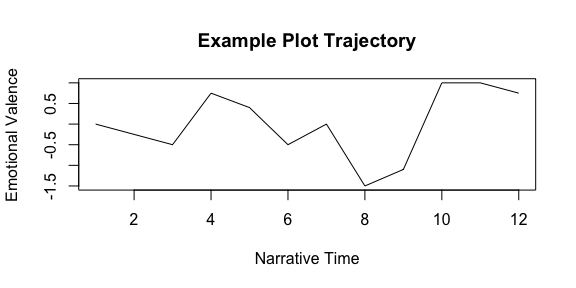With a short piece of prose, such as the one we are using in this example, the resulting plot is not very difficult to interpret. The story here begins in neutral territory, moves slightly negative and then enters a period of neutral-to-lightly-positive language. At the seventh sentence (visible between the sixth and eighth tic marks on the x-axis), however, the sentiment takes a rather negative turn downward, and reaches the low point of the passage. But after two largely negative sentences (eight and nine), the sentiment recovers with very positive tenth, eleventh, and twelfth sentences, a “happy ending” if you will.

What is observed here is useful for demonstration purposes but is hardly typical of what is seen in a 300 page novel. Over the course of three- or four-hundred pages, one will encounter quite a lot of affectual noise. Here, for example, is a plot of Joyce’s Portrait of the Artist as a Young man.

plot(
syuzhet_vector,
type="h",
main="Example Plot Trajectory",
xlab = "Narrative Time",
ylab= "Emotional Valence"
)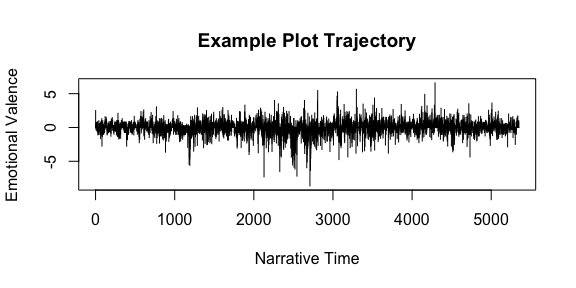While this raw data may be useful for certain applications, for visualization it is generally preferable to remove the noise and reveal the simple shape of the trajectory. One way to do that would be to apply a trend line. The next plot applies a moving average trend line to the simple example text containing twelve sentences.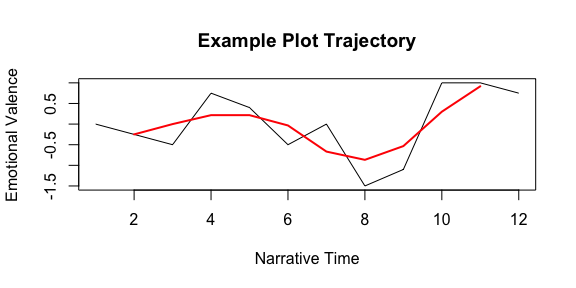While a moving average can be useful, we must remember that data on the edges is lost. Nevertheless, such smoothing can be useful for getting a sense of the emotional trajectory of a single text.

## get_percentage_values

When it comes to comparing the shape of one trajectory to another, the get_percentage_values function can be useful. The get_percentage_values function divides a text into an equal number of “chunks” and then calculates the mean sentiment valence for each. In the example below, the sentiments from Portrait are binned into 10 chunks and then plotted.

percent_vals <- get_percentage_values(syuzhet_vector, bins = 10)
plot(
percent_vals,
type="l",
main="Joyce's Portrait Using Percentage-Based Means",
xlab = "Narrative Time",
ylab= "Emotional Valence",
col="red"
)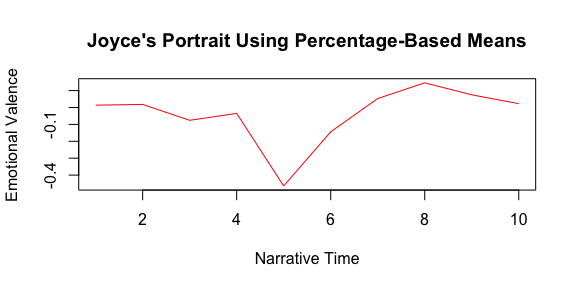Using the optional bins argument, you can control how many sentences are included inside each percentage based chunk:

percent_vals <- get_percentage_values(syuzhet_vector, bins = 20)
plot(
percent_vals,
type="l",
main="Joyce's Portrait Using Percentage-Based Means",
xlab = "Narrative Time",
ylab= "Emotional Valence",
col="red"
)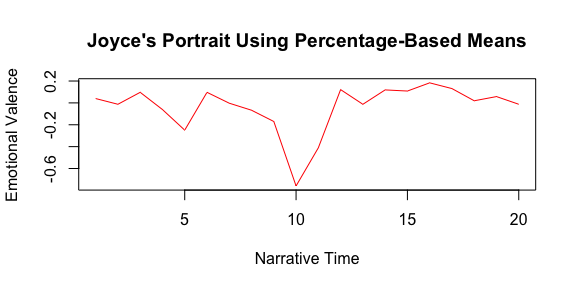Unfortunately, when a series of sentence values are combined into a larger chunk using a percentage based measure, extremes of emotional valence tend to get watered down. This is especially true when the segments of text that percentage based chunking returns are especially large. When averaged, a passage of 1000 sentences is far more likely to contain a wide range of values than a 100 sentence passage. Indeed, the means of longer passages tend to converge toward 0. But this is not the only problem with percentage-based normalization. In addition to dulling the emotional variance, percentage-based normalization makes book to book comparison somewhat futile. A comparison of the first tenth of a very long book, such as Melville’s Moby Dick with the first tenth of a short novella such as Oscar Wilde’s Picture of Dorian Grey is simply not all that fruitful because in one case the first tenth is composed of 1000 sentences and in the other just 100.

The Syuzhet package provides two alternatives to percentage-based comparison using either the Fourier or Discrete Cosine Transformations in combination with a low pass filter.

## get_transformed_values

NOTE: get_transformed_valuesis maintained for legacy purposes. Users should consider using get_dct_transform() instead.

Shape smoothing and normalization using a Fourier based transformation and low pass filtering is achieved using the get_transformed_values function as shown below. The various arguments are described in the help documentation.

library(syuzhet)
ft_values <- get_transformed_values(
syuzhet_vector,
low_pass_size = 3,
x_reverse_len = 100,
scale_vals = TRUE,
scale_range = FALSE
)
## Warning in get_transformed_values(syuzhet_vector, low_pass_size = 3,
## x_reverse_len = 100, : This function is maintained for legacy purposes.
## Consider using get_dct_transform() instead.
plot(
ft_values,
type ="l",
main ="Joyce's Portrait using Transformed Values",
xlab = "Narrative Time",
ylab = "Emotional Valence",
col = "red"
)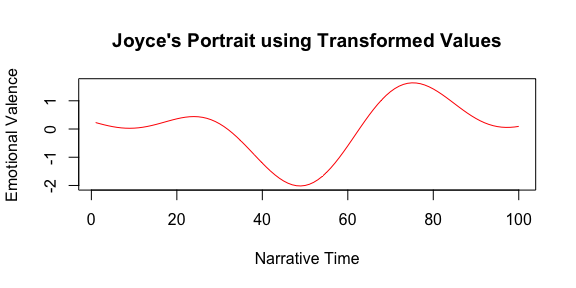## get_dct_transform

The get_dct_transform is similar to get_transformed_values function, but it applies the simpler discrete cosine transformation (DCT) in place of the fast Fourier transform. It’s main advantage is in its better representation of edge values in the smoothed version of the sentiment vector.

library(syuzhet)
dct_values <- get_dct_transform(
syuzhet_vector,
low_pass_size = 5,
x_reverse_len = 100,
scale_vals = F,
scale_range = T
)
plot(
dct_values,
type ="l",
main ="Joyce's Portrait using Transformed Values",
xlab = "Narrative Time",
ylab = "Emotional Valence",
col = "red"
)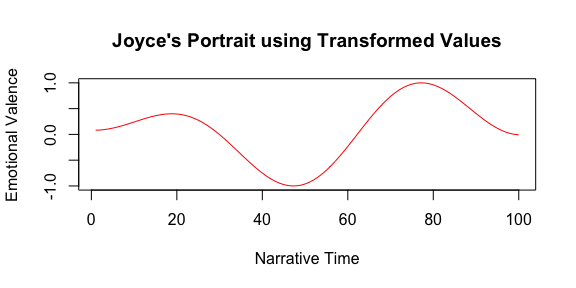## simple_plot

The simple_plot function takes a sentiment vector and applies three smoothing methods. The smoothers include a moving average, loess, and discrete cosine transformation. This function produces two plots stacked. The first shows all three smoothing methods on the same graph. The second graph shows only the DCT smoothed line, but does so on a normalized time axis. The shape of the DCT line in both the top and bottom graphs are identical. In the following code Flaubert’s Madame Bovary has been used in place of Joyce’s Portrait.

path_to_a_text_file <- system.file("extdata", "bovary.txt", package = "syuzhet")
bovary <- get_text_as_string(path_to_a_text_file)
bovary_v <- get_sentences(bovary)
bovary_sentiment <- get_sentiment(bovary_v)
simple_plot(bovary_sentiment)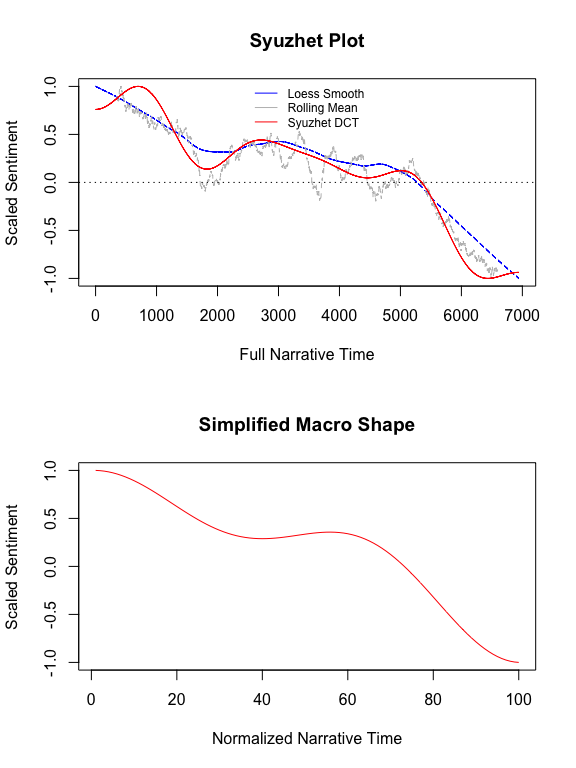## get_nrc_sentiment

The get_nrc_sentiment implements Saif Mohammad’s NRC Emotion lexicon. According to Mohammad, “the NRC emotion lexicon is a list of words and their associations with eight emotions (anger, fear, anticipation, trust, surprise, sadness, joy, and disgust) and two sentiments (negative and positive)” (See http://www.purl.org/net/NRCemotionlexicon). The get_nrc_sentiment function returns a data frame in which each row represents a sentence from the original file. The columns include one for each emotion type was well as the positive or negative sentiment valence. The example below calls the function using the simple twelve sentence example passage stored in the s_v object from above.

nrc_data <- get_nrc_sentiment(s_v)

One the data has been returned, it can be accessed as you would any other data frame. The data in the columns (anger, anticipation, disgust, fear, joy, sadness, surprise, trust, negative, positive) can be accessed individually or in sets. Here we identify the item(s) with the most “anger” and use it as a reference to find the corresponding sentence from the passage.

angry_items <- which(nrc_data$anger > 0) s_v[angry_items] ##  "I might get angry and decide to do something horrible." Likewise, it is easy to identify items that the NRC lexicon identified as joyful: joy_items <- which(nrc_data$joy > 0)
s_v[joy_items]
##  "Basically this is a very silly test."
##  "I am actually very happy today."
##  "I have finally finished writing this package."
##  "Honestly this use of the Fourier transformation is really quite elegant."
##  "You might even say it's beautiful!"

It is simple to view all of the emotions and their values:

pander::pandoc.table(nrc_data[, 1:8], split.table = Inf)
anger anticipation disgust fear joy sadness surprise trust
0 1 0 0 0 0 0 2
0 0 0 0 1 0 0 0
0 0 0 0 0 0 0 0
0 1 0 0 1 0 0 1
0 1 1 0 1 0 1 1
0 1 0 0 0 0 0 0
0 0 0 0 0 0 0 0
2 0 2 1 0 0 0 0
0 1 0 0 0 0 0 0
0 0 0 0 0 0 0 0
0 0 0 0 1 0 0 0
0 0 0 0 1 0 0 0

Or you can examine only the positive and negative valence:

pander::pandoc.table(nrc_data[, 9:10])
negative positive
0 1
1 0
1 0
0 1
0 1
0 0
0 0
2 0
0 0
0 0
0 1
0 1

These last two columns are the ones used by the nrc method in the get_sentiment function discussed above. To calculate a single value of positive or negative valence for each sentence, the values in the negative column are converted to negative numbers and then added to the values in the positive column, like this.

valence <- (nrc_data[, 9]*-1) + nrc_data[, 10]
valence
##    1 -1 -1  1  1  0  0 -2  0  0  1  1

Finally, the percentage of each emotion in the text can be plotted as a bar graph:

barplot(
sort(colSums(prop.table(nrc_data[, 1:8]))),
horiz = TRUE,
cex.names = 0.7,
las = 1,
main = "Emotions in Sample text", xlab="Percentage"
)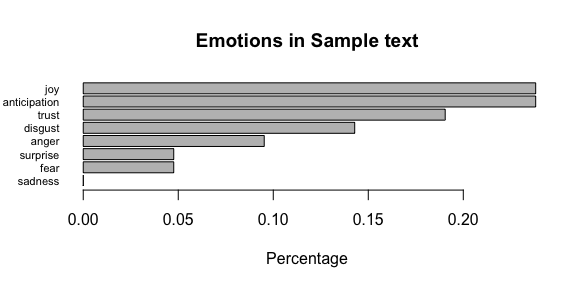## rescale_x_2

The rescale_x_2 function is handy for re-scaling values to a normalized x and y axis. This is useful for comparing two sentiment arcs. Assume that we want to compare the shapes produced by applying a moving average to two different sentiment arcs. First we’ll compute the raw sentiment values in Portrait of the Artist and Madame Bovary.

path_to_a_text_file <- system.file("extdata", "portrait.txt",package = "syuzhet")
joyces_portrait <- get_text_as_string(path_to_a_text_file)
poa_v <- get_sentences(joyces_portrait)
poa_values <- get_sentiment(poa_v, method="syuzhet")

path_to_a_text_file <- system.file("extdata", "bovary.txt", package = "syuzhet")
bovary <- get_text_as_string(path_to_a_text_file)
bovary_v <- get_sentences(bovary)
bovary_values <- get_sentiment(bovary_v)

Now we’ll calculate a moving average for each vector of raw values. We’ll use a window size equal to 1/10 of the overall length of the vector.

pwdw <- round(length(poa_values)*.1)
poa_rolled <- zoo::rollmean(poa_values, k=pwdw)
bwdw <- round(length(bovary_values)*.1)
bov_rolled <- zoo::rollmean(bovary_values, k=bwdw)

The resulting vectors are of different lengths: 4814 and 6248, so we can use rescale_x_2 to put them on the same scale.

poa_list <- rescale_x_2(poa_rolled)
bov_list <- rescale_x_2(bov_rolled)

We can then plot the two lines on the same graph even though they are of different lengths:

plot(poa_list$x, poa_list$z,
type="l",
col="blue",
xlab="Narrative Time",
ylab="Emotional Valence")
lines(bov_list$x, bov_list$z, col="red")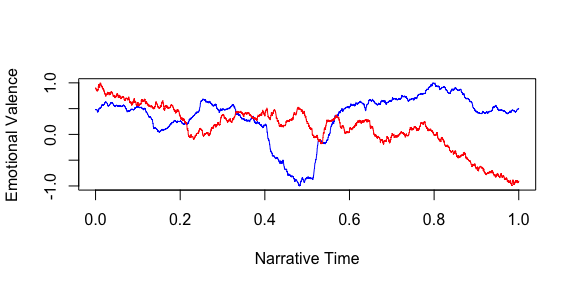Though we have now managed to scale both the x axis and y axis so as to be able to plot them on the same graph, we still don’t have vectors of the same length, which means that they cannot be easily compared mathematically. The time axis for Portrait of the Artist is 4814 units long and the time axis for Madame Bovary is 6248 units.

It is possible to sample from these vectors. In the code that follows here, we divide each vector into 100 samples and then plot those sampled points. The result is that each line is constructed out of 100 points on the x-axis.

poa_sample <- seq(1, length(poa_list$x), by=round(length(poa_list$x)/100))
bov_sample <- seq(1, length(bov_list$x), by=round(length(bov_list$x)/100))

plot(poa_list$x[poa_sample], poa_list$z[poa_sample],
type="l",
col="blue",
xlab="Narrative Time (sampled)",
ylab="Emotional Valence"
)
lines(bov_list$x[bov_sample], bov_list$z[bov_sample], col="red")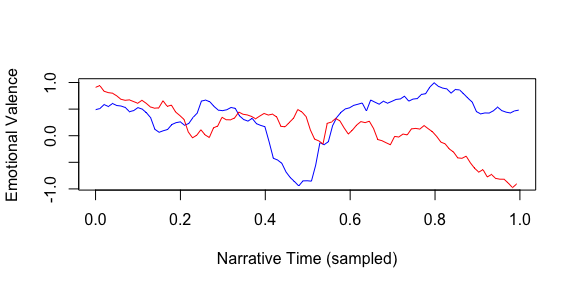With a equal number of values, in each vector, we can then apply a measure of distance or similarity, such as Euclidean distance of Pearson’s Correlation.

# Euclidean
dist(rbind(poa_list$z[poa_sample], bov_list$z[bov_sample]))
##          1
## 2 7.223739
# Correlation
cor(cbind(poa_list$z[poa_sample], bov_list$z[bov_sample]))
##            [,1]       [,2]
## [1,]  1.0000000 -0.2100283
## [2,] -0.2100283  1.0000000

Some users may find this sort of normalization and sampling preferable to the alternative method provided by the get_dct_transform, which assumes that the main flow of the sentiment trajectory is found within the low frequency components of the transformed signal. In this example, we have sampled from a set of values that have been smoothed using a moving average (which is a type of low pass filter). Once could easily apply this same routine to values that have been smoothed using some other method, such as the loess smoother that is implemented in the simple_plot function.

Here is how one might use a similar approach to sample from a loess smoothed line.

poa_x <- 1:length(poa_values)
poa_y <- poa_values
raw_poa <- loess(poa_y ~ poa_x, span=.5)
poa_line <- rescale(predict(raw_poa))
bov_x <- 1:length(bovary_values)
bov_y <- bovary_values
raw_bov <- loess(bov_y ~ bov_x, span=.5)
bov_line <- rescale(predict(raw_bov))
poa_sample <- seq(1, length(poa_line), by=round(length(poa_line)/100))
bov_sample <- seq(1, length(bov_line), by=round(length(bov_line)/100))
plot(poa_line[poa_sample],
type="l",
col="blue",
xlab="Narrative Time (sampled)",
ylab="Emotional Valence"
)
lines(bov_line[bov_sample], col="red")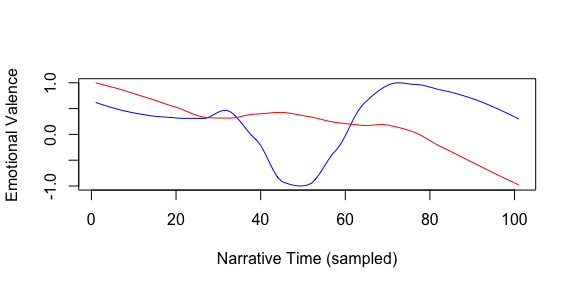## Multilingual Sentiment Lexicons

In version 2.0, support for sentiment detection in multiple languages was added by using the expanded NRC lexicon from Saif Mohammed. The lexicon includes sentiment values for 13,901 words in each of the following languages:

Arabic, Basque, Bengali, Catalan, Chinese_simplified, Chinese_traditional, Danish, Dutch, English, Esperanto, Finnish, French, German, Greek, Gujarati, Hebrew, Hindi, Irish, Italian, Japanese, Latin, Marathi, Persian, Portuguese, Romanian, Russian, Somali, Spanish, Sudanese, Swahili, Swedish, Tamil, Telugu, Thai, Turkish, Ukranian, Urdu, Vietnamese, Welsh, Yiddish, Zulu.

At the time of this release, Syuzhet will only work with languages that use Latin character sets. This effectivley means that “Arabic”, “Bengali”, “Chinese_simplified”, “Chinese_traditional”, “Greek”, “Gujarati”, “Hebrew”, “Hindi”, “Japanese”, “Marathi”, “Persian”, “Russian”, “Tamil”, “Telugu”, “Thai”, “Ukranian”, “Urdu”, “Yiddish” are not supported even though these languages are part of the extended NRC dictionary.

It is also important to point out that the sentence tokenizer inside the get_sentences() function is inherently biased for English syntax. Care should be taken to insure that the language being used is parsed properly by the get_sentences() function. It may be advisable to skip sentence tokenization in favor of word based tokenization using the get_tokens() function. Below is an example of how to call the Spanish lexicon to detect sentiment in Don Quixote.

path_to_a_text_file <- system.file("extdata", "quijote.txt",package = "syuzhet")
my_text <- get_text_as_string(path_to_a_text_file)
char_v <- get_sentences(my_text)
method <- "nrc"
lang <- "spanish"
my_text_values <- get_sentiment(char_v, method=method, language=lang)
my_text_values[1:10]
##    2  2  0  0  2  0  1 -8 -1  6

## Custom Sentiment Lexicons

In Version 2.0, functionality to allow uses to load their own custom sentiment lexicons was added to the get_sentiments() function. To work, users need to create their custom lexicon as a data frame with at least two columns named “word” and “value.” Here is a simplified example:

my_text <- "I love when I see something beautiful.  I hate it when ugly feelings creep into my head."
char_v <- get_sentences(my_text)
method <- "custom"
custom_lexicon <- data.frame(word=c("love", "hate", "beautiful", "ugly"), value=c(1,-1,1, -1))
my_custom_values <- get_sentiment(char_v, method = method, lexicon = custom_lexicon)
my_custom_values
##   2 -2

## Parallelization

Collecting sentiment results on large volumes of data can be time consuming. One could call get_sentiment and provide cluster information from parallel::makeCluster() to achieve results quicker on systems with multiple cores. For example, on Madame Bovary as above:

require(parallel)
## Loading required package: parallel
cl <- makeCluster(2) # or detect_cores() - 1
clusterExport(cl = cl, c("get_sentiment", "get_sent_values", "get_nrc_sentiment", "get_nrc_values", "parLapply"))
bovary_sentiment_par <- get_sentiment(bovary_v, cl=cl)
bovary_nrc_par <- get_sentiment(bovary_v, method='nrc', cl=cl)
stopCluster(cl)`